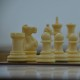guest_blog — Published On September 16, 2020 and Last Modified On September 18th, 2020

## Introduction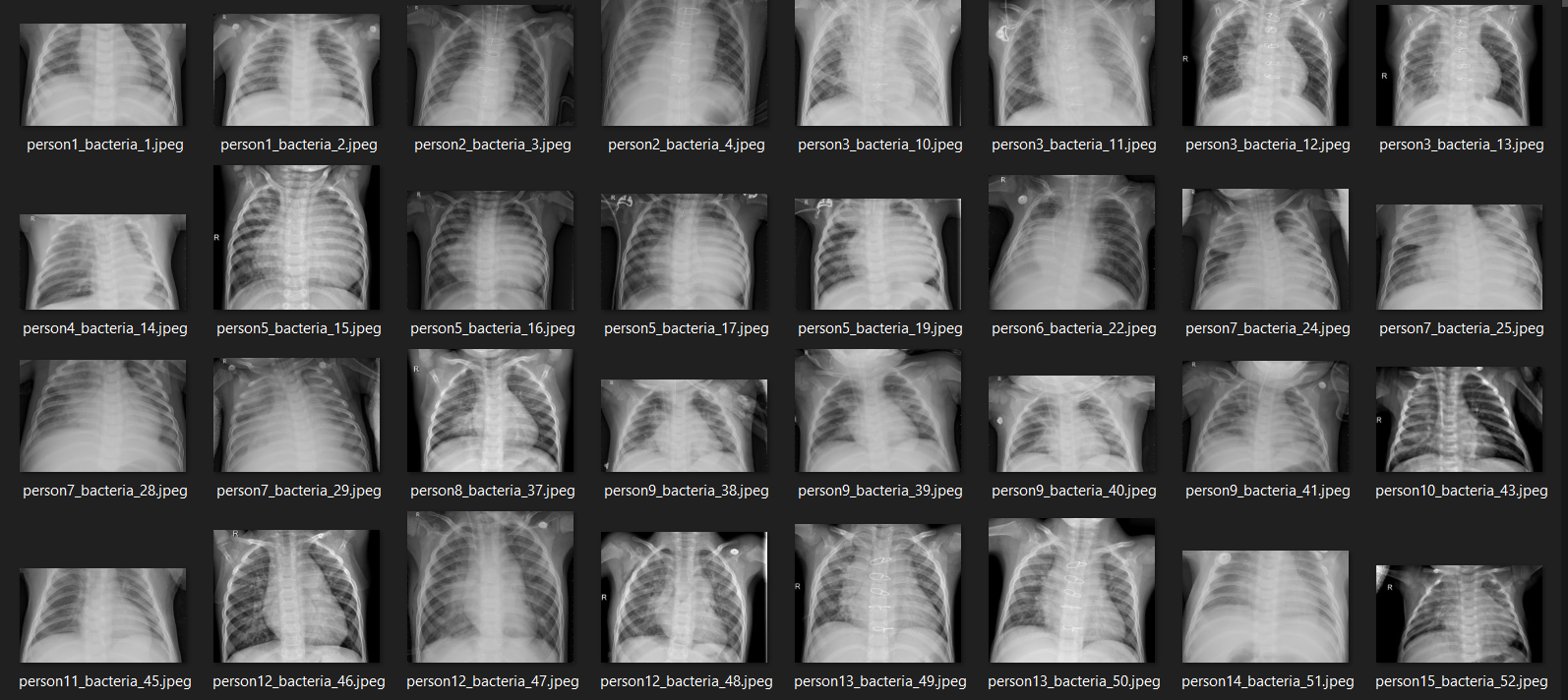Several x-ray images in the dataset used in this project.

```import os
import cv2
import pickle
import numpy as np
import matplotlib.pyplot as plt
import seaborn as sns
from tqdm import tqdm
from sklearn.preprocessing import OneHotEncoder
from sklearn.metrics import confusion_matrix
from keras.models import Model, load_model
from keras.layers import Dense, Input, Conv2D, MaxPool2D, Flatten
from keras.preprocessing.image import ImageDataGeneratornp.random.seed(22)```
```# Do not forget to include the last slash
norm_files = np.array(os.listdir(norm_path))
norm_labels = np.array(['normal']*len(norm_files))

norm_images = []
for image in tqdm(norm_files):
image = cv2.imread(norm_path + image)
image = cv2.resize(image, dsize=(200,200))
image = cv2.cvtColor(image, cv2.COLOR_BGR2GRAY)
norm_images.append(image)

norm_images = np.array(norm_images)

return norm_images, norm_labels
pneu_files = np.array(os.listdir(pneu_path))
pneu_labels = np.array([pneu_file.split('_') for pneu_file in pneu_files])

pneu_images = []
for image in tqdm(pneu_files):
image = cv2.imread(pneu_path + image)
image = cv2.resize(image, dsize=(200,200))
image = cv2.cvtColor(image, cv2.COLOR_BGR2GRAY)
pneu_images.append(image)

pneu_images = np.array(pneu_images)

return pneu_images, pneu_labels```
`norm_images, norm_labels = load_normal('/kaggle/input/chest-xray-pneumonia/chest_xray/train/NORMAL/')pneu_images, pneu_labels = load_pneumonia('/kaggle/input/chest-xray-pneumonia/chest_xray/train/PNEUMONIA/')`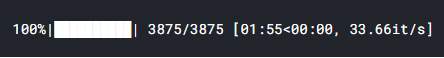The progress bar displayed using tqdm module.
```X_train = np.append(norm_images, pneu_images, axis=0)
y_train = np.append(norm_labels, pneu_labels)```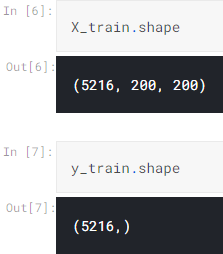The shape of the features (X) and labels (y).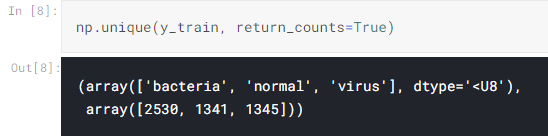Finding out the number of unique values in our training set

## Displaying several images

```fig, axes = plt.subplots(ncols=7, nrows=2, figsize=(16, 4))

indices = np.random.choice(len(X_train), 14)
counter = 0

for i in range(2):
for j in range(7):
axes[i,j].set_title(y_train[indices[counter]])
axes[i,j].imshow(X_train[indices[counter]], cmap='gray')
axes[i,j].get_xaxis().set_visible(False)
axes[i,j].get_yaxis().set_visible(False)
counter += 1
plt.show()```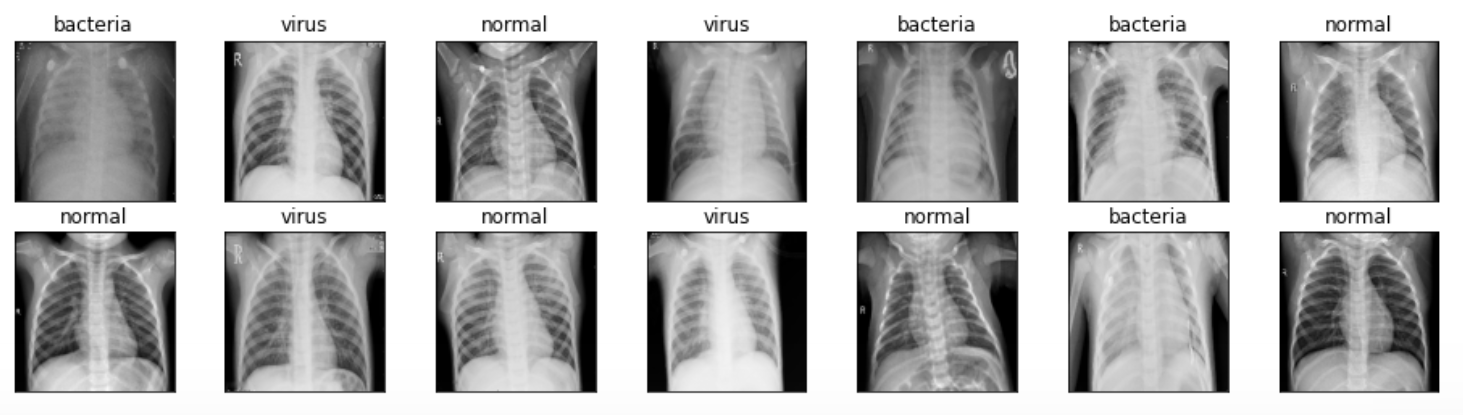Some of the preprocessed x-ray images.

```norm_images_test, norm_labels_test = load_normal('/kaggle/input/chest-xray-pneumonia/chest_xray/test/NORMAL/')pneu_images_test, pneu_labels_test = load_pneumonia('/kaggle/input/chest-xray-pneumonia/chest_xray/test/PNEUMONIA/')X_test = np.append(norm_images_test, pneu_images_test, axis=0)
y_test = np.append(norm_labels_test, pneu_labels_test)```
```# Use this to save variables
with open('pneumonia_data.pickle', 'wb') as f:
pickle.dump((X_train, X_test, y_train, y_test), f)# Use this to load variables
with open('pneumonia_data.pickle', 'rb') as f:
(X_train, X_test, y_train, y_test) = pickle.load(f)```

## Label preprocessing

```y_train = y_train[:, np.newaxis]
y_test = y_test[:, np.newaxis]```
`one_hot_encoder = OneHotEncoder(sparse=False)`
```y_train_one_hot = one_hot_encoder.fit_transform(y_train)
y_test_one_hot = one_hot_encoder.transform(y_test)```

## Reshaping X data into (None, 200, 200, 1)

```X_train = X_train.reshape(X_train.shape, X_train.shape, X_train.shape, 1)
X_test = X_test.reshape(X_test.shape, X_test.shape, X_test.shape, 1)```

## Data augmentation

```datagen = ImageDataGenerator(
rotation_range = 10,
zoom_range = 0.1,
width_shift_range = 0.1,
height_shift_range = 0.1)```
`datagen.fit(X_train)train_gen = datagen.flow(X_train, y_train_one_hot, batch_size=32)`

## CNN (Convolutional Neural Network)

```input1 = Input(shape=(X_train.shape, X_train.shape, 1))

cnn = Conv2D(16, (3, 3), activation='relu', strides=(1, 1),
cnn = Conv2D(32, (3, 3), activation='relu', strides=(1, 1),
cnn = MaxPool2D((2, 2))(cnn)

cnn = Conv2D(16, (2, 2), activation='relu', strides=(1, 1),
cnn = Conv2D(32, (2, 2), activation='relu', strides=(1, 1),
cnn = MaxPool2D((2, 2))(cnn)

cnn = Flatten()(cnn)
cnn = Dense(100, activation='relu')(cnn)
cnn = Dense(50, activation='relu')(cnn)
output1 = Dense(3, activation='softmax')(cnn)

model = Model(inputs=input1, outputs=output1)```
```model.compile(loss='categorical_crossentropy',
```history = model.fit_generator(train_gen, epochs=30,
validation_data=(X_test, y_test_one_hot))```
```Epoch 1/30
163/163 [==============================] - 19s 114ms/step - loss: 5.7014 - acc: 0.6133 - val_loss: 0.7971 - val_acc: 0.7228
.
.
.
Epoch 10/30
163/163 [==============================] - 18s 111ms/step - loss: 0.5575 - acc: 0.7650 - val_loss: 0.8788 - val_acc: 0.7308
.
.
.
Epoch 20/30
163/163 [==============================] - 17s 102ms/step - loss: 0.5267 - acc: 0.7784 - val_loss: 0.6668 - val_acc: 0.7917
.
.
.
Epoch 30/30
163/163 [==============================] - 17s 104ms/step - loss: 0.4915 - acc: 0.7922 - val_loss: 0.7079 - val_acc: 0.8045```
```plt.figure(figsize=(8,6))
plt.title('Accuracy scores')
plt.plot(history.history['acc'])
plt.plot(history.history['val_acc'])
plt.legend(['acc', 'val_acc'])
plt.show()plt.figure(figsize=(8,6))
plt.title('Loss value')
plt.plot(history.history['loss'])
plt.plot(history.history['val_loss'])
plt.legend(['loss', 'val_loss'])
plt.show()```

## Model evaluation

```predictions = model.predict(X_test)
predictions = one_hot_encoder.inverse_transform(predictions)```
`cm = confusion_matrix(y_test, predictions)`
```classnames = ['bacteria', 'normal', 'virus']plt.figure(figsize=(8,8))
plt.title('Confusion matrix')
sns.heatmap(cm, cbar=False, xticklabels=classnames, yticklabels=classnames, fmt='d', annot=True, cmap=plt.cm.Blues)
plt.xlabel('Predicted')
plt.ylabel('Actual')
plt.show()```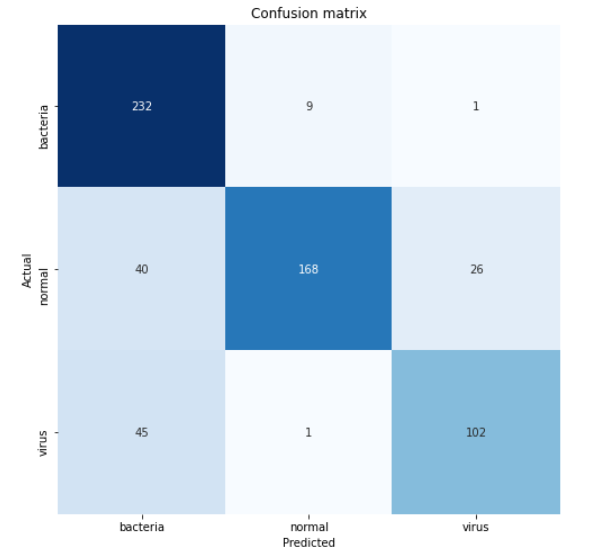Confusion matrix constructed based on test data.
```import os
import cv2
import pickle	# Used to save variables
import numpy as np
import matplotlib.pyplot as plt
import seaborn as sns
from tqdm import tqdm	# Used to display progress bar
from sklearn.preprocessing import OneHotEncoder
from sklearn.metrics import confusion_matrix
from keras.models import Model, load_model
from keras.layers import Dense, Input, Conv2D, MaxPool2D, Flatten
from keras.preprocessing.image import ImageDataGenerator	# Used to generate images

np.random.seed(22)

# Do not forget to include the last slash
norm_files = np.array(os.listdir(norm_path))
norm_labels = np.array(['normal']*len(norm_files))

norm_images = []
for image in tqdm(norm_files):
image = cv2.imread(norm_path + image)
# Resize image to 200x200 px
image = cv2.resize(image, dsize=(200,200))
# Convert to grayscale
image = cv2.cvtColor(image, cv2.COLOR_BGR2GRAY)
norm_images.append(image)

norm_images = np.array(norm_images)

return norm_images, norm_labels

pneu_files = np.array(os.listdir(pneu_path))
pneu_labels = np.array([pneu_file.split('_') for pneu_file in pneu_files])

pneu_images = []
for image in tqdm(pneu_files):
image = cv2.imread(pneu_path + image)
# Resize image to 200x200 px
image = cv2.resize(image, dsize=(200,200))
# Convert to grayscale
image = cv2.cvtColor(image, cv2.COLOR_BGR2GRAY)
pneu_images.append(image)

pneu_images = np.array(pneu_images)

return pneu_images, pneu_labels

# All images are stored in _images, all labels are in _labels
norm_images, norm_labels = load_normal('/kaggle/input/chest-xray-pneumonia/chest_xray/train/NORMAL/')
pneu_images, pneu_labels = load_pneumonia('/kaggle/input/chest-xray-pneumonia/chest_xray/train/PNEUMONIA/')

# Put all train images to X_train
X_train = np.append(norm_images, pneu_images, axis=0)

# Put all train labels to y_train
y_train = np.append(norm_labels, pneu_labels)

print(X_train.shape)
print(y_train.shape)
# Finding out the number of samples of each class
print(np.unique(y_train, return_counts=True))

print('Display several images')
fig, axes = plt.subplots(ncols=7, nrows=2, figsize=(16, 4))

indices = np.random.choice(len(X_train), 14)
counter = 0

for i in range(2):
for j in range(7):
axes[i,j].set_title(y_train[indices[counter]])
axes[i,j].imshow(X_train[indices[counter]], cmap='gray')
axes[i,j].get_xaxis().set_visible(False)
axes[i,j].get_yaxis().set_visible(False)
counter += 1
plt.show()

# Do the exact same thing as what we have done on train data
norm_images_test, norm_labels_test = load_normal('/kaggle/input/chest-xray-pneumonia/chest_xray/test/NORMAL/')
pneu_images_test, pneu_labels_test = load_pneumonia('/kaggle/input/chest-xray-pneumonia/chest_xray/test/PNEUMONIA/')
X_test = np.append(norm_images_test, pneu_images_test, axis=0)
y_test = np.append(norm_labels_test, pneu_labels_test)

# Save the loaded images to pickle file for future use
with open('pneumonia_data.pickle', 'wb') as f:
pickle.dump((X_train, X_test, y_train, y_test), f)

# Here's how to load it
with open('pneumonia_data.pickle', 'rb') as f:
(X_train, X_test, y_train, y_test) = pickle.load(f)

print('Label preprocessing')

# Create new axis on all y data
y_train = y_train[:, np.newaxis]
y_test = y_test[:, np.newaxis]

# Initialize OneHotEncoder object
one_hot_encoder = OneHotEncoder(sparse=False)

# Convert all labels to one-hot
y_train_one_hot = one_hot_encoder.fit_transform(y_train)
y_test_one_hot = one_hot_encoder.transform(y_test)

print('Reshaping X data')
# Reshape the data into (no of samples, height, width, 1), where 1 represents a single color channel
X_train = X_train.reshape(X_train.shape, X_train.shape, X_train.shape, 1)
X_test = X_test.reshape(X_test.shape, X_test.shape, X_test.shape, 1)

print('Data augmentation')
# Generate new images with some randomness
datagen = ImageDataGenerator(
rotation_range = 10,
zoom_range = 0.1,
width_shift_range = 0.1,
height_shift_range = 0.1)

datagen.fit(X_train)
train_gen = datagen.flow(X_train, y_train_one_hot, batch_size = 32)

print('CNN')

# Define the input shape of the neural network
input_shape = (X_train.shape, X_train.shape, 1)
print(input_shape)

input1 = Input(shape=input_shape)

cnn = Conv2D(16, (3, 3), activation='relu', strides=(1, 1),
cnn = Conv2D(32, (3, 3), activation='relu', strides=(1, 1),
cnn = MaxPool2D((2, 2))(cnn)

cnn = Conv2D(16, (2, 2), activation='relu', strides=(1, 1),
cnn = Conv2D(32, (2, 2), activation='relu', strides=(1, 1),
cnn = MaxPool2D((2, 2))(cnn)

cnn = Flatten()(cnn)
cnn = Dense(100, activation='relu')(cnn)
cnn = Dense(50, activation='relu')(cnn)
output1 = Dense(3, activation='softmax')(cnn)

model = Model(inputs=input1, outputs=output1)

model.compile(loss='categorical_crossentropy',

# Using fit_generator() instead of fit() because we are going to use data
# taken from the generator. Note that the randomness is changing
# on each epoch
history = model.fit_generator(train_gen, epochs=30,
validation_data=(X_test, y_test_one_hot))

# Saving model
model.save('pneumonia_cnn.h5')

print('Displaying accuracy')
plt.figure(figsize=(8,6))
plt.title('Accuracy scores')
plt.plot(history.history['acc'])
plt.plot(history.history['val_acc'])
plt.legend(['acc', 'val_acc'])
plt.show()

print('Displaying loss')
plt.figure(figsize=(8,6))
plt.title('Loss value')
plt.plot(history.history['loss'])
plt.plot(history.history['val_loss'])
plt.legend(['loss', 'val_loss'])
plt.show()

# Predicting test data
predictions = model.predict(X_test)
print(predictions)

predictions = one_hot_encoder.inverse_transform(predictions)

print('Model evaluation')
print(one_hot_encoder.categories_)

classnames = ['bacteria', 'normal', 'virus']

# Display confusion matrix
cm = confusion_matrix(y_test, predictions)
plt.figure(figsize=(8,8))
plt.title('Confusion matrix')
sns.heatmap(cm, cbar=False, xticklabels=classnames, yticklabels=classnames, fmt='d', annot=True, cmap=plt.cm.Blues)
plt.xlabel('Predicted')
plt.ylabel('Actual')
plt.show()```

## References

### About the AuthorA computer science undergraduate student of Universitas Gadjah Mada, Yogyakarta, Indonesia. I’m a big fan of the machine and deep learning stuff.

### About the Author## 2 thoughts on "Pneumonia Detection using CNN with Implementation in Python"###### ernest says:September 19, 2020 at 9:09 pm
where is the github repo for the source code? Reply